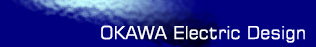# Feedback system using a Transfer Function Analysis and Design Tool

This page is a web application that determine transfer function from a feedback system. The transfer function is simulated frequency analysis and transient analysis on graphs, showing Bode diagram, Nyquist diagram, Impulse response and Step response.

The transfer function of feedback system is the following general equation.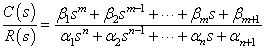Transient analysis condition is nm. maximum n=12

### Example

At a given the following compensation

 G(s)= 0.0001s2+10s+10000 s

 H(s)= 1e8 s2+1000s+1e8

The following form is an example to input G(s) and H(s). Use the right form to simulate.
Gβ=
Gα=

Hβ=
Hα=

Select the following Systems: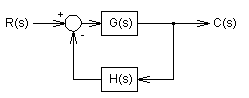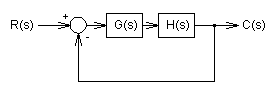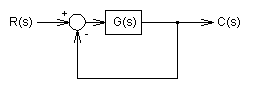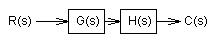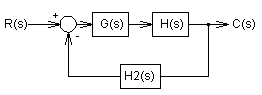Gβ=
Gα=

Hβ=
Hα=

H2β=
H2α=

### Frequency analysis

Bode diagram
Phase  Group delay
Nyquist diagram(f=0→∞)
Pole, zero
Phase margin
Oscillation analysis

Upper and lower frequency limits:
f1= - f2=[Hz] (frequency limits are optional)

### Transient analysis

Step response
Impulse response
Overshoot
Final value of the step response

Simulation time:
0 - [sec] (optional)